# 计算机视觉技术在Kaggle数据集上的应用• 这里是来自Fast.ai库的一个中等大小的玩具数据集，
• 这里是来自Fast.ai库的大型玩具数据集，
• 在此导出外部数据集，
• 在这里通过网络搜索创建我们自己的数据集。

A.如何在Colab中使用Kaggle数据集，以及

B.如何使用Fast.ai快速手册的第07课中介绍的归一化、调整大小和测试时间放大计算机视觉技术。Lesson 07 in the Fast.ai FastBook

A.在Colab中使用Kaggle数据集

a.如果您还没有帐户，请创建一个。它是免费的，而且处理得很快。

b.探索您感兴趣的数据集/比赛。这个博客涉及鸟类的计算机视觉/图像分类。computer vision/ images classification for birds

c.为每个类选择至少包含20幅图像的数据集。否则，您可能会遇到错误。

d.我已经能够对图像使用.jpg和.png格式。如果遇到.tfrec格式，请查找指向原始数据集的链接。

e.在安装前完成此操作。如果您进行了设置并分心，笔记本运行可能会断开连接，您需要重新运行。

2.设置。

a.笔记本

b.安装和进口

i.Fast.ai库的一般设置。

!pip install -Uqq fastbookimport fastbookfastbook.setup_book()from fastbook import *#!pip install fastai -U  # unhash if this is your first useimport fastaifrom fastai.vision.all import *

• 在Kaggle页面中，打开您的“帐户”。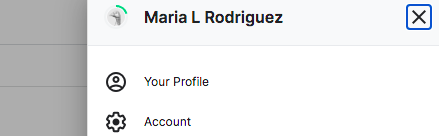• 创建新的API令牌。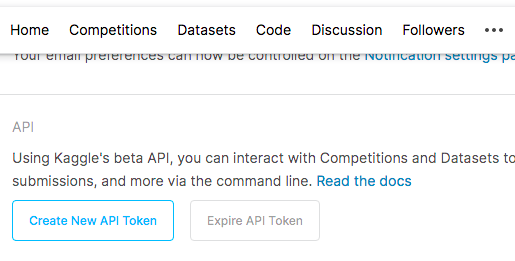• 在Colab笔记本中，安装Kaggle并上传API Token/Kaggle json文件。

!pip install -q kagglefrom google.colab import filesfiles.upload()

• 创建Kaggle目录并启用访问。

!mkdir ~/.kaggle!cp kaggle.json ~/.kaggle/

3.收集您的数据。

a.下载代码格式。

• ！卡格尔
• 数据集还是竞赛集(例如，在Kaggle中，您的集合是来自数据集还是竞赛部分？)
• 下载
• 路径

!kaggle datasets download 'gpiosenka/100-bird-species/train'

b.从下载的压缩文件中获取单个文件，并在完成后删除该压缩文件。

!unzip \*zip && rm *.zip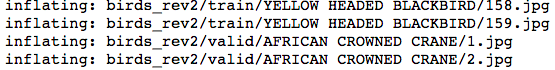c.指定路径。

train_path = 'birds_rev2/train'

B.高级成像技术示例。

dblock = DataBlock(    (ImageBlock(), CategoryBlock()),    get_items = get_image_files,    get_y = parent_label,    splitter = RandomSplitter(seed=42),    item_tfms = Resize(460),    batch_tfms = aug_transforms(size=224))dls = dblock.dataloaders(train_path)dls.train.show_batch()dls.train.show_batch(unique=True)model = xresnet50(n_out = dls.c)learn_base = Learner(dls, model, loss_func = CrossEntropyLossFlat(),                     metrics = accuracy)learn_base.fit_one_cycle(5, 3e-3)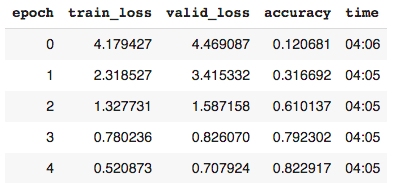• Learner是Fast.ai中的代码类，用于组装数据、模型和培训。
• Xresnet是一种顺序的、非预先训练的神经网络。
• n_out=dls.c表示类别或标签的数量。
• 交叉熵损失是模型能够学习的计算。它是从类的预测概率导出的。概率值的范围从0到1。当这些值被转换为交叉熵时，接近0(非常差的预测)的概率变得更加明显，因此受到的惩罚更多。
• 交叉熵损失平坦是交叉熵损失的重组，便于处理。
• 虽然计算机需要损失提供的梯度信息，但是精度度量更好地服务于人的解释。

learn_base.lr_find()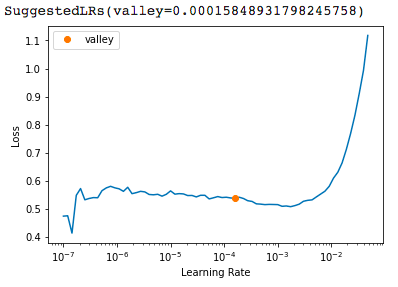learn_base.show_results()2.应用归一化变换。

dblock = DataBlock(      (ImageBlock, CategoryBlock),      get_items = get_image_files,      get_y = parent_label,      item_tfms = Resize(460),      batch_tfms = [*aug_transforms(size=224),                    Normalize.from_stats(*imagenet_stats)]) #dls_norm = dblock.dataloaders(train_path, bs=64)

x, y = dls.one_batch()          # baselinexn, yn = dls_norm.one_batch()   # normalized

print('Non-normalized tensors:', x[:10])print('Mean:',x.mean(dim = [0,2,3]))print('Std:', x.std(dim = [0,2,3]))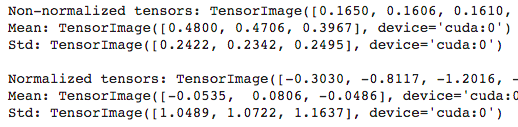model = xresnet50(n_out = dls_norm.c)learn_norm = Learner(dls_norm, model, loss_func =    CrossEntropyLossFlat(), metrics = accuracy)learn_norm.fit_one_cycle(5, 3e-3)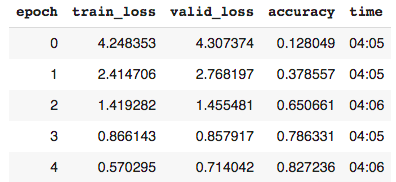3.应用渐进式大小调整

dblock = DataBlock(      (ImageBlock, CategoryBlock),      get_items = get_image_files,      get_y = parent_label,      item_tfms = Resize(460),      batch_tfms = aug_transforms(size=128)) # start smalldls_128 = dblock.dataloaders(train_path, bs=64)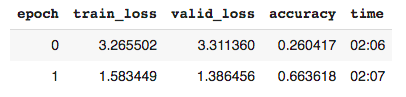dblock = DataBlock(      (ImageBlock, CategoryBlock),      get_items = get_image_files,      get_y = parent_label,      item_tfms = Resize(460),      batch_tfms = aug_transforms(size=224)) # biggerdls_224 = dblock.dataloaders(train_path, bs=64)

learn_128.dls = dls_224learn_128.fit_one_cycle(3, 3e-3)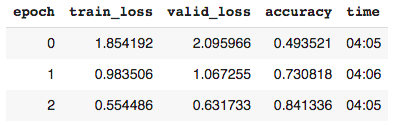4.使用测试时间增加(TTA)。

# using the baseline dblock and dlsmodel = xresnet50(n_out = dls.c)learn = Learner(dls, model,                loss_func = CrossEntropyLossFlat(),                metrics = accuracy)

5.结合这些先进的转换技术。

dblock = DataBlock( (ImageBlock, CategoryBlock), get_items = get_image_files, get_y = parent_label, item_tfms = Resize(460), batch_tfms = aug_transforms(size=128))  # dls_128 = dblock.dataloaders(train_path, bs=64)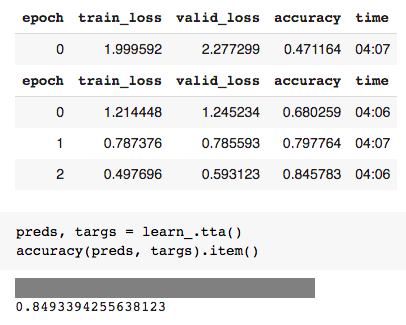interp = ClassificationInterpretation.from_learner(learn_)interp.most_confused(min_val = 5)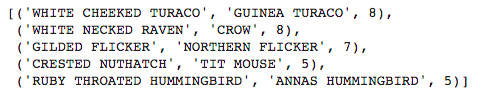6.让我们一起玩吧！

btn_upload = widgets.FileUpload()btn_upload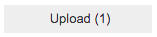img = PILImage.create(btn_upload.data[-1])out_pl = widgets.Output()out_pl.clear_output()with out_pl: display(img.to_thumb(250))pred, pred_idx, probs = learn_.predict(img) # revlbl_pred = widgets.Label()lbl_pred.value = f'Prediction: {pred}; Probability: {probs[pred_idx]:.04f}'btn_run = widgets.Button(description = 'Classify')btn_run